Next: 2.1.2 The BCS Penetration Up: 2.1 The Magnetic Penetration Previous: 2.1 The Magnetic Penetration

## 2.1.1 The London Penetration Depth

Let us first consider the magnetic penetration depth in the context of the conventional London theory  at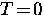. If a magnetic field is applied to a superconductor which is initially in zero field, the magnetic field is a function of time. According to the Maxwell equation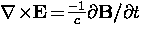,the time-varying magnetic field gives rise to an electric field. In a normal metal this will induce eddy currents, but in a superconductor the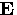-field will give rise to persistent currents (i.e. supercurrents). The induced supercurrents will in turn generate a magnetic field of their own which opposes the applied magnetic field. If the applied magnetic field is weak, the flux is totally screened from the bulk of the superconductor. This phenomenon is often described as perfect diamagnetism''. From Newton's law, the equation of motion for a superconducting carrier with mass m and charge -e in the presence of an electric fieldis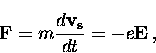(6)
where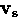is the velocity of the superconducting carrier. The field-induced supercurrent density is given by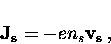(7)
where ns is the local density of superconducting carriers. Substituting Eq. (2.7) into Eq. (2.6) gives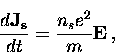(8)
which is known as the first London equation''. Taking the curl of both sides of Eq. (2.8) gives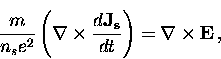(9)
which can be rewritten using the Maxwell equation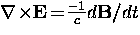to give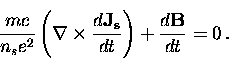(10)
In order to obtain the Meissner effect (i.e.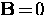in the bulk of the superconductor) the London brothers removed the time derivative in Eq. (2.10) and postulated the new equation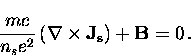(11)
Equation (2.11) is commonly referred to as the second London equation''. Since the supercurrent density is related to the field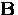by another Maxwell equation(12)
substitution of Eq. (2.12) into Eq. (2.11) gives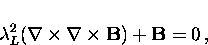(13)
where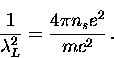(14)
The variable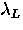is called the London penetration depth. Note that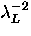is proportional to the superfluid density ns.

The relationship betweenandis best realized by a simple example. Consider the vacuum-superconductor interface illustrated in Fig. 2.1. If a field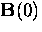is applied parallel to the surface of the superconductor, the solution of Eq. (2.13) is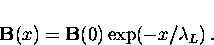(15)
Thus, both the magnetic field B(x) and the supercurrent density Js(x) decay exponentially with distance inside the superconductor over the length scale.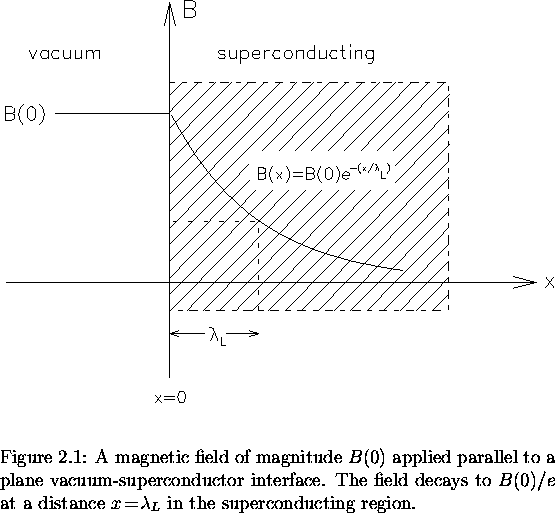Equation (2.14) was derived for.At nonzero temperature the behaviour ofcan be approximated by incorporating the two-fluid model of Gorter and Casimir . In this model the electron system is assumed to contain a superconducting component with electron density ns, and a normal component with an electron density nn. The total electron density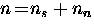becomes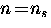at, and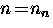for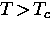, where Tc is the superconducting transition temperature. For arbitrary temperature, Gorter and Casimir found that good agreement with early experiments could be obtained if one assumes that.When this expression is combined with Eq. (2.14) the penetration depth is given by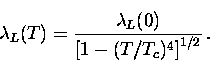(16)
As the temperature T increases, ns is reduced and the magnetic penetration depth increases with T, such that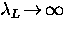as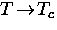.Although this relation gives a good first-order description of many of the experimental results for conventional superconductors, the temperature dependence is not a prediction from the two-fluid model. Better agreement is obtained with the behaviour predicted from the microscopic theory developed by Bardeen, Cooper and Schrieffer (BCS) .

Before considering the BCS theory, let us work backwards to determine the kernel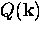in Eq. (2.2) for the London theory. The second London equation [i.e. Eq. (2.13)] can be rewritten using the Maxwell relation in Eq. (2.12) and the relation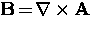to give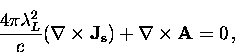(17)
which is easily solved for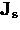to give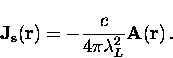(18)
The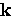th Fourier component ofis thus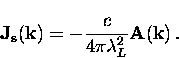(19)
Comparing Eq. (2.19) with Eq. (2.2) gives the following simple result for the London kernel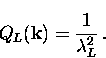(20)
Clearly, QL is independent of. This implies that the London theory is a local theory, where the value ofat a point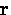only depends on the value of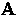at that same point.Generally, the value ofin the vicinity of the point whereis being measured is also important. In a more general nonlocal theory,depends on.

The current density due to the normal electrons is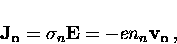(21)
where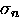is the finite conductivity of the normal fluid. Thus, the total current density at arbitrary temperature below Tc is simplyNext: 2.1.2 The BCS Penetration Up: 2.1 The Magnetic Penetration Previous: 2.1 The Magnetic Penetration